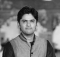## How to add class to an element without addClass() method in jQuery?AmitDiwan

Updated on 10-Apr-2023 14:49:19

We can use the .attr() method to add a class to an element. This method can be used to set or get the value of an attribute on an HTML element. To add a class, we first select the element using a jQuery selector, and then call the .attr() method, ... Read More

## Computing the Cartesian Product of Two Sets in JavaScriptAmitDiwan

Updated on 31-Mar-2023 15:40:15

Cartesian Product Inset theory a Cartesian product is a mathematical operation that returns a set (or product set or simply product) from multiple sets.That is, for sets A and B, the Cartesian product A × B is the set of all ordered pairs (a, b) where a ∈ A and b ... Read More

## Computing the Cartesian Product of Multiple Arrays in JavaScriptAmitDiwan

Updated on 31-Mar-2023 15:39:43

We are required to write a JavaScript function that takes in multiple arrays of numbers. The function should return an array of the cartesian product of the elements from all the arrays.For example −If the input arrays are −[1, 2], [10, 20], [100, 200, 300]Then the output should be −const ... Read More

## JavaScript Program to Generate all rotations of a numberAmitDiwan

Updated on 15-Mar-2023 15:20:03

We will generate all rotations of a number in JavaScript by converting the number into a string, then rotating the characters of the string, and finally converting it back into a number. This process will be repeated for each rotation. We will continuously repeat this process until we have generated ... Read More

## JavaScript Program to Generate a matrix having sum of secondary diagonal equal to a perfect squareAmitDiwan

Updated on 15-Mar-2023 15:17:28

We will write a JavaScript program that generates a matrix with the sum of the secondary diagonal being a perfect square. Our program will use nested loops to traverse the matrix and calculate the sum of the secondary diagonal elements. We will then use the Math.sqrt() method to find the ... Read More

## JavaScript Program to Form coils in a matrixAmitDiwan

Updated on 15-Mar-2023 15:15:13

We will be using JavaScript to form coils in a matrix. The process involves manipulating the elements of the matrix to create a spiral pattern. This can be achieved by changing the direction of traversal, keeping track of the visited elements, and adjusting the indices accordingly. We will be continuously ... Read More

## JavaScript Program to Find the subarray with least averageAmitDiwan

Updated on 15-Mar-2023 15:13:18

We are going to write a program that will find the subarray with the least average. To do this, we will iterate through the array and keep track of the current subarray and its sum. For each element, we will calculate the average of the current subarray and compare it ... Read More

## JavaScript Program to Find the Longest Bitonic Subsequence | DP-15AmitDiwan

Updated on 15-Mar-2023 15:10:30

We will be finding the longest bitonic subsequence in each array using dynamic programming. A bitonic subsequence is a sequence which is first increasing, then decreasing. To find the longest bitonic subsequence, we will use a two-step approach. First, we will find the longest increasing subsequence in the given array, ... Read More

## JavaScript Program to find simple interestAmitDiwan

Updated on 15-Mar-2023 15:06:07

We will use the formula for simple interest to calculate the interest for a given principal amount, rate of interest, and time period. Simple interest is calculated as the product of the principal amount, rate of interest, and time period. This formula makes it easy for us to find the ... Read More

## JavaScript program to find perimeter of a triangleAmitDiwan

Updated on 15-Mar-2023 15:03:49

We will find the perimeter of a triangle by adding the lengths of its three sides. To do this, we will first declare three variables to store the lengths of the sides and then use the addition operator to sum these values and store the result in a fourth variable. ... Read More